# Статьи журнала - Вестник Южно-Уральского государственного университета. Серия: Математическое моделирование и программирование

Все статьи: 679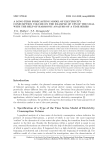A long-term forecasting model of electricity consumption volume on the example of UPS of the Ural with the help of harmonic analysis of a time series

Mokhov V.G., Demyanenko T.S.

Краткое сообщение

In this article, the model of forecasting of electricity consumption volume is analyzed on the basis of harmonic analysis of a time series. Previously, we establish the presence of a trend component described by a second-order polynomial. Based on the construction of the autocorrelation function, the periodicity of the time series of electricity consumption volume is proved, with periods equal to 1 year and 1 week, due to decrease in electricity consumption in summer because of increase in daylight hours and decrease in production on weekends. The model is tested on actual hourly data of United Energy System of the wholesale market for electricity and power in Russia. The model is tested for adequacy using Fisher's criterion and the coefficient of determination. The introduction of two harmonic components (annual and weekly ones) instead of the generally accepted one reduces the approximation error for the current model from 2,25 % to 2,08 %, which provides increased energy efficiency for consumers while reducing the fines of the balancing market. The proposed scientific tools are recommended in the entity's operating activity of electric power to forecast the major energy market parameters in order to reduce the fines by improving the accuracy of forecasts.

Бесплатно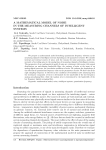A mathematical model of noise in the measuring channels of intelligent systems

Volovich G.I., Solomin E.V., Topolskaya I.G., Topolsky D.V.

Статья научная

We propose a mathematical model describing quantization frequency selection in the measuring channels of intelligent systems depending on the parameters of noise. We consider internal and external sources of noise, seek the reasons for noise generation, justify the necessity of recording noise in the analog lines of measuring channels of intelligent systems. The parameters of our mathematical model of noise are: stationary white noise with normal distribution, an anti-aliasing bandwidth filter, the variance of noise at the input of an analog-digital converter, the correlation coefficient of noise. We evaluate the dependence of the selection of quantization frequency value on the parameters of noise. We show that if the right sampling frequency is selected in the conditions of broadband noise measuring then the systematic component of error is determined by the bandwidth of the low frequency analog anti-aliasing filter, while the random error is determined by the bandwidth of the low frequency digital averaging filter.

Бесплатно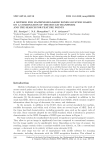A method for machine-readable zones location based on a combination of the Hough transform and the search for feature points

Savelyev B.I., Skoryukina N.S., Arlazarov V.V.

Статья научная

This article describes a method for machine-readable zones location in document images based on a combination of the Hough transform and the search for feature points. The search for feature points, filtering, and clustering using the Hough transform are described step-by-step. In addition to the machine-readable zone location, we develop a solution for determining the orientation of the zone. This method is designed to meet the requirements for real-time operation on mobile devices. The paper presents the results of measuring the quality of the method on an open synthetic dataset and the operating time on mobile devices. An experimental study on an artificial dataset show that the proposed algorithm allows to achieve a quality of 0,82 in terms of the mean value of the Jaccard indices. The operating time of the proposed algorithm for machine-readable zone location on a mobile device is 6 ms on the iPhone SE 2.

Бесплатно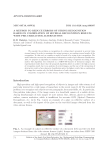A method to reduce errors of string recognition based on combination of several recognition results with per-character alternatives

Bulatov K.B.

Статья научная

We consider the problem on recognition of a string object presented in several video stream frames. In order to maximize the output accuracy, we combine several results of the recognition. To this end, we consider a model of result of a string object recognition. The model takes into account the estimations of alternative results of per-character classification. Also, we propose an algorithm to combine results of a string recognition according to this model. The algorithm was evaluated on a MIDV-500 dataset of document images. The experimental results show that the proposed algorithm allows to achieve the high accuracy of recognition result due to an analysis of several images, and the use of the estimations of alternative results of per-character classification gives the higher results then a combination of strings that contain only the final alternatives of each character.

Бесплатно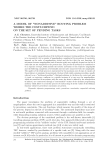A model of "nonadditive" routing problem where the costs depend on the set of pending tasks

Chentsov A.G., Salii Ya.V.

Статья обзорная

We consider a generalization of the bottleneck (minimax) routing problem. The problem is to successively visit a number of megalopolises, complicated by precedence of constraints imposed on the order of megalopolises visited and the fact that the cost functions (of movement between megalopolises and of interior tasks) may explicitly depend on the list of tasks that are not completed at the present time. The process of movement is considered to be a sequence of steps, which include the exterior movement to the respective megalopolis and the following completion of (essentially interior) jobs connected with the megalopolis. The quality of the whole process is represented by the maximum cost of steps it consists of; the problem is to minimize the mentioned criterion (which yields a minimax problem, usually referred to as a "bottleneck problem"). Optimal solutions, in the form of a route-track pair (a track, or trajectory, conforms to a specific instance of a tour over the megalopolises, which are numbered in accordance with the route; the latter is defined by the transposition of indices), are constructed through a "nonstandard" variant of the dynamic programming method, which allows to avoid the process of constructing of all the values of the Bellman function whenever precedence constraints are present.

Бесплатно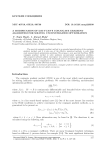A modification of Dai-Yuan’s conjugate gradient algorithm for solving unconstrained optimization

Najm Huda Y., Ahmed Huda I.

Краткое сообщение

The spectral conjugate gradient method is an essential generalization of the conjugate gradient method, and it is also one of the effective numerical methods to solve large scale unconstrained optimization problems. We propose a new spectral Dai-Yuan (SDY) conjugate gradient method to solve nonlinear unconstrained optimization problems. The proposed method's global convergence was achieved under appropriate conditions, performing numerical testing on 65 benchmark tests to determine the effectiveness of the proposed method in comparison to other methods like the AMDYN algorithm and some other existing ones like Dai-Yuan method.

Бесплатно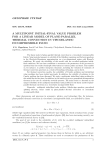A multipoint initial-final value problem for a linear model of plane-parallel thermal convection in viscoelastic incompressible fluid

Zagrebina S.A.

Статья обзорная

The linear model of plane-parallel thermal convection in a viscoelastic incompressible Kelvin-Voigt material amounts to a hybrid of the Oskolkov equations and the heat equations in the Oberbeck-Boussinesq approximation on a two-dimensional region with Bénard's conditions. We study the solvability of this model with the so-called multipoint initial-final conditions. We use these conditions to reconstruct the parameters of the processes in question from the results of multiple observations at various points and times. This enables us, for instance, to predict emergency situations, including the violation of continuity of thermal convection processes as a result of breaching technology, and so forth. For thermal convection models, the solvability of Cauchy problems and initial-final value problems has been studied previously. In addition, the stability of solutions to the Cauchy problem has been discussed. We study a multipoint initial-final value problem for this model for the first time. In addition, in this article we prove a generalized decomposition theorem in the case of a relatively sectorial operator. The main result is a theorem on the unique solvability of the multipoint initial-final value problem for the linear model of plane-parallel thermal convection in a viscoelastic incompressible fluid.

Бесплатно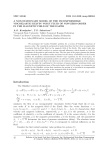A non-stationary model of the incompressible viscoelastic Kelvin-Voigt fluid of non-zero order in the magnetic field of the Earth

Kondyukov A.O., Sukacheva T.G.

Статья научная

We investigate the Cauchy-Dirichlet problem for a system of Oskolkov equations of nonzero order. The considered mathematical model describes the flow of an incompressible viscoelastic Kelvin-Voigt fluid in the magnetic field of the Earth. The model takes into account that the fluid is subject to various external influences, which depend on both the coordinate of the point in space and the time. The first part of the paper presents the known results obtained by the authors earlier and based on the theory of solvability of the Cauchy problem for semilinear nonautonomous Sobolev type equations. In the second part, we reduce the considered mathematical model to an abstract Cauchy problem. In the third part, we prove the main result that is the theorem on the existence and uniqueness of the solution. Also, we establish the conditions for the existence of quasi-stationary semitrajectories, and describe the extended phase space of the model under study. In this paper, we summarize our results for the Oskolkov system that simulates the motion of a viscoelastic incompressible Kelvin-Voigt fluid of zero order in the magnetic field of the Earth.

Бесплатно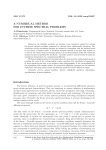A numerical method for inverse spectral problems

Статья научная

Basing on the Galerkin methods, we develop a new numerical method for solving the inverse spectral problems generated by discrete lower semibounded operators. The restrictions on the perturbing operator are relaxed in comparison with the method based on the theory of regular traces. A Fredholm integral equation of the first kind enables us to recover the values of the perturbing operator at the discretization nodes. We tested the method on spectral problems for the Sturm - Liouville operator, and the results of numerous simulations demonstrate its computational efficiency. We found simple formulas for the eigenvalues of a discrete lower semibounded operator avoiding the roots of the corresponding secular equations. The calculation of eigenvalues of these operators can start at an arbitrary index independently of the (un)availability of the eigenvalues with smaller indices. For perturbed selfadjoint operators we can calculate eigenvalues with large indices when the Galerkin method becomes difficult to apply.

Бесплатно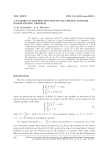A numerical method for solving quadratic integer programming problem

Tatyankin V.M., Shitselov A.V.

Статья научная

We propose a new numerical method for solving quadratic integer programming problem. The algorithm is based on a special representation of a minimizer of the corresponding objective functional. The problem can be reduced to a special box-constrained integer least squares problem. The advantage of the proposed algorithm is a good computational performance (approximately O(nln(n))operations) shown in numerical experiments, where the number of unknowns can be up to 108. The computational complexity of the algorithm is confirmed experimentally by a large number of numerical experiments. The algorithm consists of 3 steps. At the average, a solution is found at the second step in 83,6% cases, while the third step gives solution in the remaining cases. The algorithm is realized with the use of the Python programming language. The results of numerical experiments can be found at the service GitHubGist. The elaborated software system was used to solve the problem on formation of the optimal order for education institutions in regions of the Russian Federation.

Бесплатно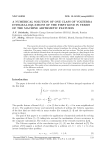A numerical solution of one class of Volterra integral equations of the first kind in terms of the machine arithmetic features

Solodusha S.V., Mokry I.V.

Статья научная

The research is devoted to a numerical solution of the Volterra equations of the first kind that were obtained using the Laplace integral transforms for solving the equation of heat conduction. The paper consists of an introduction and two sections. The first section deals with the calculation of kernels from the respective integral equations at a fixed length of the significand in the floating point representation of a real number. The PASCAL language was used to develop the software for the calculation of kernels, which implements the function of tracking the valid digits of the significand. The test examples illustrate the typical cases of systematic error accumulation. The second section presents the results obtained from the computational algorithms which are based on the product integration method and the midpoint rule. The results of test calculations are presented to demonstrate the performance of the difference methods.

Бесплатно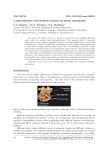A parametric stochastic model of bone geometry

Zalyapin V.I., Timofeev Yu.S., Shishkina E.A.

Статья научная

The aim of the present study is to develop a parametric bone modelling algorithm which takes into account bone microarchitecture. This approach allows to generate hematopoietic bone segment phantoms based on literature-derived micro - and macro dimensions. We propose a method for subdividing bones into small segments which can be described by simple geometric shapes filled with a stochastically generated rod-like model of the trabecular structure with appropriate voxel resolution. This approach avoids the disadvantages of non-parametric individual modelling based on computer tomography scans. The parametric method allows the simulation of individual variability in bone-specific dimensions. The model presented in this paper will be used to describe the geometry of hematopoietic sites, which in turn will serve as a basis for calculating the doses of irradiation of the hematopoietic cells of the bone marrow from the incorporated beta-emitters.

Бесплатно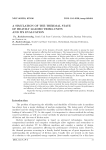A simulation of the thermal state of heavily loaded tribo-units and its evaluation

Статья научная

The thermal state of the elements of heavily loaded tribo-units is among the most important parameters affecting their performance. The temperature of the lubricating layer of bearings determines to a large extent their load-bearing capacity. The heat balance equation used to estimate the temperature of friction pairs fails to yield the temperature fields and the regions of their maximum values. This fact makes our problem important. We examine a mathematical model and a method for calculating the thermal state and thermohydrodynamic characteristics of heavily loaded sliding bearings, taking into account the non-Newtonian properties of the fluid as well as the heat exchange processes between the lubricating layer and the surrounding moving surfaces of tribo-units. To solve the energy equation, we propose to use finite difference approximation methods. To create the difference analogs of the energy equations for some structural elements and thin lubricant layers, we use the Pismen-Reckford scheme of implicit alternating directions. We present the calculated hydromechanical characteristics of the connecting rod bearing of a heat engine. We obtain three-dimensional distributions of temperature in the lubricant. The results show that, if we allow for convective heat transfer in the radial direction, the processes of heat exchange between the lubricating layer and the surrounding moving surfaces enable us to determine more accurately the mean lubricant temperature and the thermal stress of a tribo-unit as a whole. Our method can be used to assess the performance and efficiency of heavily loaded tribo-units of piston and rotary machines.

Бесплатно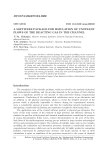A software package for simulation of unsteady flows of the reacting gas in the channel

Gidaspov V.Yu., Golubev V.K., Severina N.S.

Статья научная

This paper describes a software package for numerical modelling of the structure of unsteady flows of multicomponent reacting gas with graphic and informational components for resource-intensive phases of computational experiments support. Simulation of the "fine structure" of unsteady flows is achieved because the calculation is carried out at substantially irregular mobile computational mesh, including the fact that the trajectories of strong and weak discontinuities, the parameters of which are calculated by special grid-characteristic algorithms, serve as computational knots; herewith all the gridline intersections are calculated precisely. The designed software package can be used to solve the problems of reacting gas dynamic, which may have practical significance, as well as to illustrate academic courses in physical gas dynamics.

Бесплатно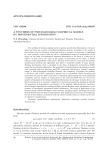A synthesis of pseudo-Boolean empirical models by precedential information

Donskoy V.I.

Статья научная

The problem of decision-making based on partial, precedential information is the most important when the creation of artificial intelligence systems. According to the results of observations over the behaviour of external objects or systems it is necessary to synthesize or, more precisely, extract from the data a mathematical model of optimization of the object on the basis of accumulated empirical information in the form of a finite set of triples: "a state vector, the value of the quality of functioning of the object, a binary indicator of the admissibility of this state". The aim of the work is to create and substantiate mathematical methods and algorithms that allow to synthesize models of scalar pseudo-Boolean optimization with a constraint in the form of disjunctive normal form (DNF) using this precedential information. The peculiarity of pseudo-Boolean optimization models with separable objective functions and DNF constraint, which has a bounded constant length, is their polynomial solvability. However, the complexity of bringing the problem to the form with a DNF constraint in general case is exponential. When extracting the model from the data the DNF constraint is synthesized approximately but with polynomial complexity and the number of conjunctions in the extracted DNF does not exceed the number of examples in the initial precedential information. In the paper is shown how to use binary decision trees to construct a disjunctive constraint, proposed the methods to identify the properties of monotonicity and linearity of the partially defined objective functions, and developed algorithms for solving problems pseudo-Boolean scalar optimization in the presence of incomplete, precedential initial information. The scope of application of the obtained results includes intelligent control systems, intelligent agents. Although the control models derived from the data are approximate, their application can be more successful than the use of less realistic, inconsistent with the objects models which are chosen on the base of subjective considerations.

Бесплатно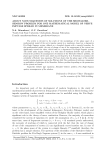About nonuniqueness of solutions of the Showalter-Sidorov problem for one mathematical model of nerve impulse spread in membrane

Manakova N.A., Gavrilova O.V.

Краткое сообщение

The article is devoted to the study of the morphology of the phase space of a mathematical model of the nerve impulse spread in a membrane, based on a degenerate Fitz Hugh-Nagumo system, defined on a bounded domain with a smooth boundary. In this mathematical model, the rate of change of one of the components of the system can significantly exceed the other, which leads to a degenerate Fitz Hugh-Nagumo system. The model under inquiry belongs to a wide class of semilinear Sobolev type models. To research the problem of nonuniqueness of solutions of the Showalter-Sidorov problem, the phase space method will be used, which was developed by G.A. Sviridyuk to scrutinize the solvability of Sobolev type equations. We have shown that the phase space of the studied model contains singularity such as the Whitney fold. The conditions of existence, uniqueness or multiplicity of solutions of the Showalter-Sidorov problem depending on the parameters of the system are found.

Бесплатно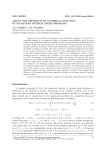About one approach to numerical solution of nonlinear optimal speed problems

Buldaev A.S., Burlakov I.D.

Статья научная

Optimal speed problems are among the most important problems of the theory of controlled systems. In the qualitative theory of nonlinear speed problems one of the main results is the Pontryagin maximum principle. For the numerical solution of nonlinear speed problems, along with methods based on the maximum principle, methods of reducing to auxiliary problems of optimal control using linearization, parameterization, discretization, and other techniques are widely used. The complexity of numerical methods is determined by the number of iterations to find a solution to the speed problem with a given accuracy. A universal computational procedure that is effective for calculating a variety of speed problems does not currently exist. Therefore, it is actual to develop special approaches to reduce the amount of calculations and reduce the number of iterations. The paper proposes a new approach based on the reduction of a nonlinear speed problem to an auxiliary optimization problem with mixed control functions and parameters. To search for a solution to the emerging auxiliary problem, a specially developed form of conditions for nonlocal improvement of admissible control in the form of a fixed-point problem of the control operator, and a constructed iterative algorithm for successive improvement of admissible controls are used. Approbation and comparative analysis of the computational efficiency of the proposed fixed point approach is carried out on known models of optimal speed problems.

Бесплатно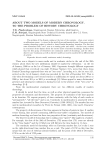About two models of modern chronology. To the problem of history chronology

Plyshevskaya T.K., Buriyak E.M.

Краткое сообщение

The problem of the dispute, arising at the turn of the centuries - when a new century or millennium comes - is viewed in the article from mathematical point. Controversy about starting chronology points is related to Anno Domini chronology invention. The fact that some researchers took "zero" year as a starting point and others - the first year, resulted in occurrence of the dispute about the correct choice of modern chronology. Authors deem that the first group of researchers use continuous model of chronology, and the other - discreet one. Continuous as well as discreet models are used in modern life in different field of activity.

Бесплатно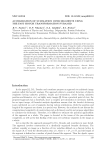Acceleration of summation over segments using the fast Hough transformation pyramid

Soshin K.V., Nikolaev D.P., Gladilin S.A., Ershov E.I.

Статья научная

In this paper, we propose an algorithm for fast approximate calculation of the sums over arbitrary segments given by a pair of pixels in the image. Using the results of intermediate calculations of the fast Hough transform, the proposed algorithm allows to calculate the sum over arbitrary line segment with a logarithmic complexity depending on the linear size of the original image (also called fast discrete Radon transform or Brady transform). In this approach, the key element of the algorithm is the search for the dyadic straight line passing through two given pixels. We propose an algorithm for solving this problem that does not degrade the general asymptotics. We prove the correctness of the algorithm and describe a generalization of this approach to the three-dimensional case for segments of straight lines and of planes.

Бесплатно

Журнал